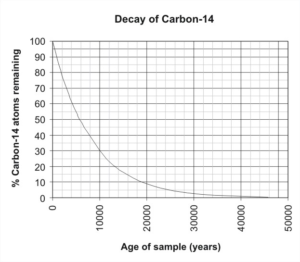## Putting It Together: Exponential and Logarithmic Equations and Models

At the start of this module, you were assigned the task of analyzing a fossilized bone to determine its age. To make that estimate, you need to model for the decay rate of carbon-14.

The decay of a radioactive element is an exponential function of the form:

$A\left(t\right)=A_0e^{-kt}$

where

$A(t)$ = mass of element remaining after t years

$A_0$ = original mass of element

$k$ = rate of decay

$t$ = time in years

So to create a model for the decay function of carbon-14, assume for simplicity that the sample you started with had a mass of 1g. We know that half the starting mass of the sample will remain after one half-life which is 5,730 years. We can substitute these values for $A(t)$ and $A_0$ as follows:

$A\left(t\right)=A_0e^{-kt}$

$\frac{1}{2}=(1)e^{-k\left(5730\right)}$

$1n\left(\frac{1}{2}\right)=1n\left(e^{-k\left(5730\right)}\right)$

$1n\left(2^{-1}\right)=\left(-5730k\right)1n\left(e\right)$

$-1n\left(2\right)=-5730k\left(1\right)$

$k\approx1.21\times10^{-4}$

Now you know the decay rate so you can write the equation for the exponential decay of carbon-14 and you can represent it as a graph.The next step is to evaluate the function for a given mass. Assume a starting mass of 100 grams and that there are 20 grams remaining. Substitute these values into the model in the following way:

 Write the equation $A(t)=100e^{\large{-(0.000121)t}}$ Substitute 20 grams for A(t) $20=100e^{\large{-\left(0.000121\right)t}}$ Divide both sides by 100 $0.20=e^{\large{-\left(0.000121\right)t}}$ Change to logarithmic form $1n\left(0.20\right)=-\left(0.000121\right)t$ Divide both sides by -0.000121 $t={\large\frac{1n\left(0.20\right)}{-0.000121}}$ Solve $t\approx13,301$ years

Now you know that it would take 13,301 years for a 100-gram sample of carbon-14 to decay to the point that only 20 grams are left. Confirm that this number makes sense by looking at the graph.

You can also determine the amount of a 100-gram sample that would remain after a given number of years such as 8,000.  To do this, substitute the number of years into the function and evaluate.

$A\left(t\right)=100e^{-\left(0.000121\right)t}$

$A\left(8000\right)=100e^{-\left(0.000121\right)\left(8000\right)}\approx38$ grams

About 38 grams would remain after 8,000 years.

Understanding exponential functions helps scientists better understand radioactive decay and provides insights into past civilizations and species.